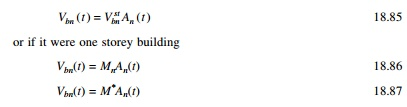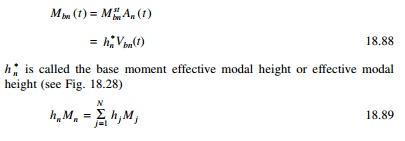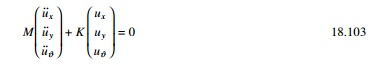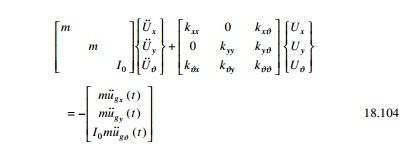Home | | Structural Dynamics and Earthquake Engineering | Effective modal mass and modal height

# Effective modal mass and modal height

base shear modal mass or brevity effective modal mass.

Effective modal mass and modal height

The base shear for ŌĆśnŌĆÖ mode is calculated asM n* is called base shear modal mass or brevity effective modal mass. From that it is clear that only the portion of Mn of the mass of a multi-storey building is effective in producing base shear due to nth mode because the building mass is distributed among various floor levels and the equivalent static force mjŽĢjn varies over the height. The sum of effective modal masses Mn over the modes is equal to the mass of this building.Example 18.11

Determine the effective modal mass and the effective modal height for the frame shown in Fig. 18.29.

Solution

The modal distribution of effective earthquake force is given in Fig. 18.30. Taking moment at baseThe effective modal mass and modal height are indicated in Fig. 18.31.

Example 18.12

Consider a five storey building (Example 18.1) (see Fig. 18.32) whose properties are given. Calculate effective modal mass and height.The effective modal mass and modal height are shown in Fig. 18.34.

The ground acceleration u╦Ö╦Ög (t ) is defined by its numerical value as time instant equally spaced at Ōłåt. This time step is chosen small enough to define u╦Ö╦Ög ( t ) and to determine accurately the response of the SDOF system:

Base shear = m (7.0926A1(t) + 0.6471A2(t) + 0.2A3(t)

+ 0.0528A4(t) + 0.0003A5(t))

Base moment = mh (7.0927 ├Ś 3.079A1(t) + 0.6471 ├Ś (ŌĆō1.563)A2(t)

+ 0.2A3(t) + 0.0528 ├Ś (ŌĆō0.587)A4(t)

0.0003 ├Ś 7.67A5(t))

Multiple support excitation

There are certain examples in which the ground motion generated by an earthquake is different from support to support. For example the Golden Gate Bridge is 1965 m in length and the ground motion is expected to vary significantly over the length of the base at the two ends of the bridge.

Example18.13

A uniform two span continuous bridge shown in Fig. 18.35 with flexural stiffness EI idealized as lumped mass. Let us formulate the equation of motion subjected to vertical motion at 1, 2, 3 as ug1, ug2, ug3 at supports.

Formulation stiffness matrix 10 ├Ś 10. Assuming translational displacement as master and other degrees of freedom as slaves we get reduced stiffness matrix of size 5 ├Ś 5.

Apply unit load at 3 (to get influence vector i1)

Moment at 4 = L

Calculate u2 when u3 = 1

The ŌĆō sign shown the deflection at 2 is downward.

Now calculate deflection at 1 due to unit load at 3= deflection at 3 due to unit load at 1.

Both deflections at 3 and 1 are upwards and hence positive. If u3 = 1 let us calculate what is u1

To find the influence vector i2, apply unit load at 4 and find the deflection at 1 and 4. The deflection at 4 due to unit load at 4 is given by L3/6EI. If the load is at a distance of ├Ś2 and the deflection is to be calculated at ├Ś1, then deflection at ├Ś1 is given

When the deflection at 4 is equal to 1 what is the deflection at 1 and 2 which may be calculated as

Symmetric plan buildings: translational ground motion

Consider an N-storey symmetric plan building having rigid floor displacement and several frames in each x and y direction as shown in Fig. 18.36.1 One storey, two way unsymmetric system

Consider the idealized one storey frame shown in Fig. 18.37. Assume the diaphragm is rigid. Assume frame A is located at a distance of e:2 Equation of motionConsidering earthquake excitation defined by u╦Ö╦Ögx ( t ), u╦Ö╦Ögy ( t ), u╦Ö╦Ög╬Ė ( t ) , we get the equation of motion if (kxC = kxB)The above equations are coupled. Thus the response of the system to x and y components of ground motion is not restricted to lateral displacement x and y directions but will also include lateral motions in tranverse directions and the torsion of the roof diaphragm about the vertical axis.

In Fig. 18.36, if frame A passes through the centre of mass ŌĆśOŌĆÖ, then (e = 0) (kxB = kxC).All three equations are uncoupled and solved.

Study Material, Lecturing Notes, Assignment, Reference, Wiki description explanation, brief detail
Civil : Structural dynamics of earthquake engineering : Effective modal mass and modal height |